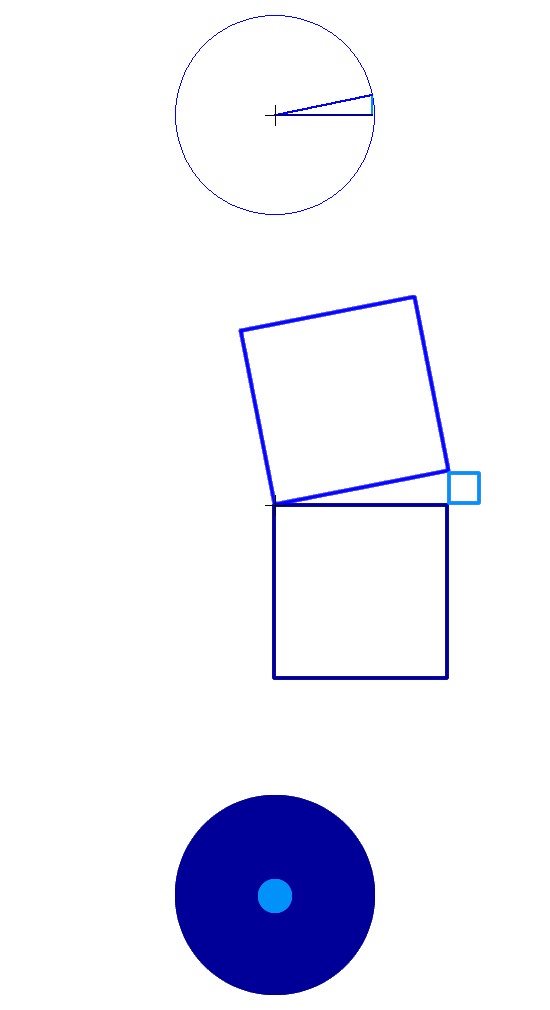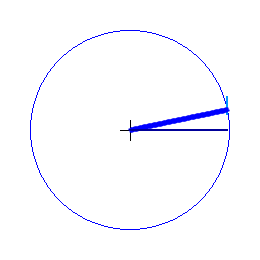## Light wave/particle duality recap

In science light rays are at the same time waves and particles. In the famous 2 slits experiments, light waves, which ocupy large volumes of space, pass through 2 parallel slits at the same time, interfering with themselves on the other side and forming interferences; individual particle photons, which like particle matter have extremely small volumes, sent through the same slits should not provide interferences, yet they do.
Light waves and particle photons cannot make one, hence the label: light duality.

Based on physics mathematics, gravimotion's interpretation conciliates photon and wave.

## Uncovering the photon's real entity

In science a photon is defined as being energy E provided by Planck's equation E = h . f . Energy is a quality that can be transferred to an object in order to perform work, or provide heat; h is Planck's constant and f is the frequency characterising the photon considered, defined as a number of cycles per second.

In gravimotion, a photon cycle (the very word used in number of cycles per second) is the period in seconds of the wave (Note 1) defined by the frequency f; for instance, a photon of frequency f = 10 periods per second, is a photon which cycle is occurring in .1 second that is a period = .1 second. Mathematically f = 1 / period duration (a time in seconds) that is the inverse of a time.
Keeping the very same letter f for symbol (Note 2), the photon energy remains E = h . f , the letter f representing now the inverse of a time duration.

The photon's period occurring in time is a reality that must also be occurring in space along its wavelength; a photon is a wave truncated to one of its cycles.
In gravimotion interpretation of Nature, photons' so called particles occurring as individual wave's period/wavelength cycles, are behaving just as waves do.

## The photon constitution

In this animation, single photons are popping out of your screen one after another and reach your eyes; each is occurring as a motion-volume precisely defined as a cylinder having for base the surface area of the top circle and for length the time you see it, which is a duration determined by the full revolution of the blue radius; a duration precisely equal to the wave's period that is a repetitive cycle (Note 3).

Beware, such photons / motion-volumes are not part of physics's science; here these motion-volumes are oscillations of the present mo(ve)ment; it nevertheless mimicks the Quantum Field theory, as physical motion is now replacing the Quantum field.

In science physics the electric and magnetic fields of any electromagnetic wave are represented with two sinusoids offset 900 degrees with respect to each other; the important point though is when either one of the two fields is null the other is maximum or minimum, as such the wave can also be represented as a sine and a cosine events occurring simultaneously.
The animations below are coinciding to a specific polarized light ray, such as a blue photon for instance, but represented at a much lower frequency.In the top animation the plain blue revolving radius of length r determines successive angles; the cosines of these angles (the successive horizontal dark blue lines) coincide to the electric field variation; the sines (the vertical light blue lines) coincide to the magnetic field; as can be seen the electric (horizontal cosine lines) and magnetic (vertical sine lines) fields are at 90 degrees with respect to each other just as interpreted in science physics. Usually the sine is represented coincident to the vertical axle; here, for the reason explained next, the sine values are shifted to the end of the cosines.

In the second animation, squares are attached to these 3; according to Pythagorean's theorem the area of the radius squared is at all times equal to the sum of the coinciding horizontal line length squared (cosine squared) and the vertical line length squared (sine squared); in this illustration the 3 radius, cosine and sine lengths have been multiplied by √ Π (read square root of pie) so the surfaces of the squares attached to the revolving radius (in plain blue) are at all times precisely equal to the surface of above circle; the light and dark blue squares areas only are varying.
In gravimotion the electric-magnetic duality occurs as follows: the electric component (dark blue squares) below the horizontal to start with, while decreasing is transferring its energy to the magnetic (light blue squares) above the horizontal, which as a consequence is increasing; when this magnetic component reaches a maximum it in turn rebounds within the common allocated motion-volume, returning back its energy to the electric component; a full cycle is involving 4 transfers.

The third animation illustrates this interaction; the dark blue surface (cosine squared or electric field) and the lightblue surface (sine squared or magnetic field) are mutually interacting with each other, ending up as a photon's motion-volume as defined in the first paragraph of this section.

Shown next is how the electric to magnetic back and forth mutual interaction is carrying out the wave's very moving reality.

## The photon's motion

In gravimotion interpretation, the present mo(ve)ment (science's space-time) is assumed to be at the image of the air molecules motions surrounding us out of which the material molecules have been removed and within which light waves rather than sound waves are travelling (see Space-time or present mo(ve)ment); the photon's are occurring as well defined oscillations of the present mo(ve)ment, the latter occuring under motion-volumes in all directions.In this illustration, rather than popping out of the screen and travelling toward your eyes, the photons are travelling left to right; it highlights the fact that when the electric field is maximum (outside perimeter dark blue) it is also moving fast in space that is browsing great distances in little time; on the other hand, when the magnetic field is maximum (outside perimeter light blue) the photon motion-volume is taking long time durations to cover short distances. In this illustration, as above, the photon's motion is greatly slowed down to highlight this intertwining.
Furthermore the back and force electric to magnetic volumes synergy is interacting with the un-coordinated motion-volumes sea within which it is progressing, actually modifying the latter along and producing the photon's overall linear motion; at the image of your own walking, in which the energy propelling you is your very own body acting on the outside ground. A process opposite the concept of force, which is instead an independent entity of the object it is moving, and which is applied from outside that object to make it move.
The direction of the motion-volumes, while maintained as is unless modified, is determined by the emitter or the source of the oscillation.

## Present mo(ve)ment (spacetime) constitution

In the absence of the electromagnetic wave energy, the above plain blue radiuses rather than oscillating in harmony have erratic angular orientations implementing the present mo(ve)ment (space time).Because they are exclusively horizontal, the dark blue cosine line's orientations may be considered to be variations occurring along a trajectory on Earth; the light blue sine line's orientations perpendicular to that trajectory are covering no ground and as such varying in time only.
The absence of electric and magnetic oscillations, unveils space and time tight intertwin.
Even though advocated by Einstein's space-time, this space/time/oneness is difficult to imagine as most of us human beings think that time is running independently of anything else and more so independently of matter and space. How could time be part of stones and metal objects? And how could time be part of space?
The way to answer is to note that the present only is real; to morrow even the next second do not occur; and yesterday along last second are no longer; everything that surrounds us in space along the whole material Universe, the latter being integral part of space, is constantly renewing itself along the present, the past just as the future never in force.

The present mo(ve)ment, the Universe's fabric, is a reality in which time and space blend.

This space/time oneness has dire consequences; now space and the present's duration make one; this allows for two essential functions of Nature that are: first keeping the identity of things alive and second allowing their evolution; these two in turn are mandating that space and time duration be overlapping themselves rather than time occurring in sequences and independently of space.

## Fascinating but not satisfying

While Planck's E = h f equation is eloquent, the assertion that such particles cannot produce fringes has no foundation. As a matter of fact experiments show that particles emitted one at a time do exhibit fringes pattern.
Besides identifying the photon to the wave, gravimotion's verbiage does not provide explanation to the duality phenomenon; such identification may be then considered as displeasing and as unsatisfactory as the photon point particle is.
I am fascinated; the reason for my writing is it might be of interest to other human minds who would then be able to clarify the situation.

## Notes:

Note 1 : A cycle could as well be chosen to be a wavelength, as in De Broglie relation, which involves momentum instead of energy; in that case the number of cycles per second becomes a speed.

Note 2 : As a matter of coincidence, flip upside down the letter f in your mind and you get a t.

Note 3 : Gravimotion's photon very short time duration complies with science's mathematics just as science's hypothetical frequency does comply with reality.

Copyright © 2021 Henri Salles. You have the permission to reproduce, print, distribute and post the contents of this website, provided you mention the link: https://gravimotion.com. Click to Contact us.